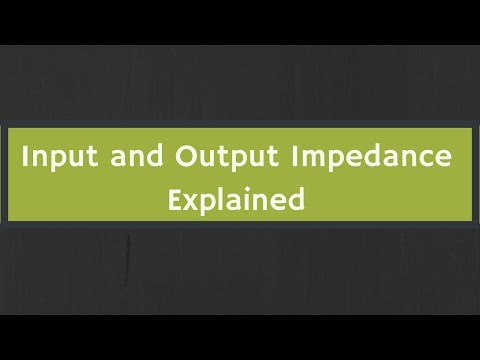# Blog

## What is the input impedance of a transistor?## Is there a formula for output impedances and output resistance?

• There do not appear to be any simple formulas for the output impedances. However, R. Victor Jones develops expressions for output resistance. [RVJ] Amplifier characteristics, adapted from GE Transistor Manual, Figure 1.21.

## What are the different types of impedances in an amplifier?

• A common theoretical model has total 4 impedances for an amplifier: Input impedance, output impedance, transfer impedance and feedback impedance. Those impedances are complex numbers in equations which describe how input signal current and voltage depend on each other and on signal current and voltage in output.

## Can a transistor be used as an amplifier?

• Transistor is a common part which can act as an amplifier. To design a proper circuit around a transistor to make a working amplifier one needs those theoretical impedances. The impedances depend on frequency and on the applied dc voltages and currents.The manufacturer publishes them in the datasheet of the transistor.